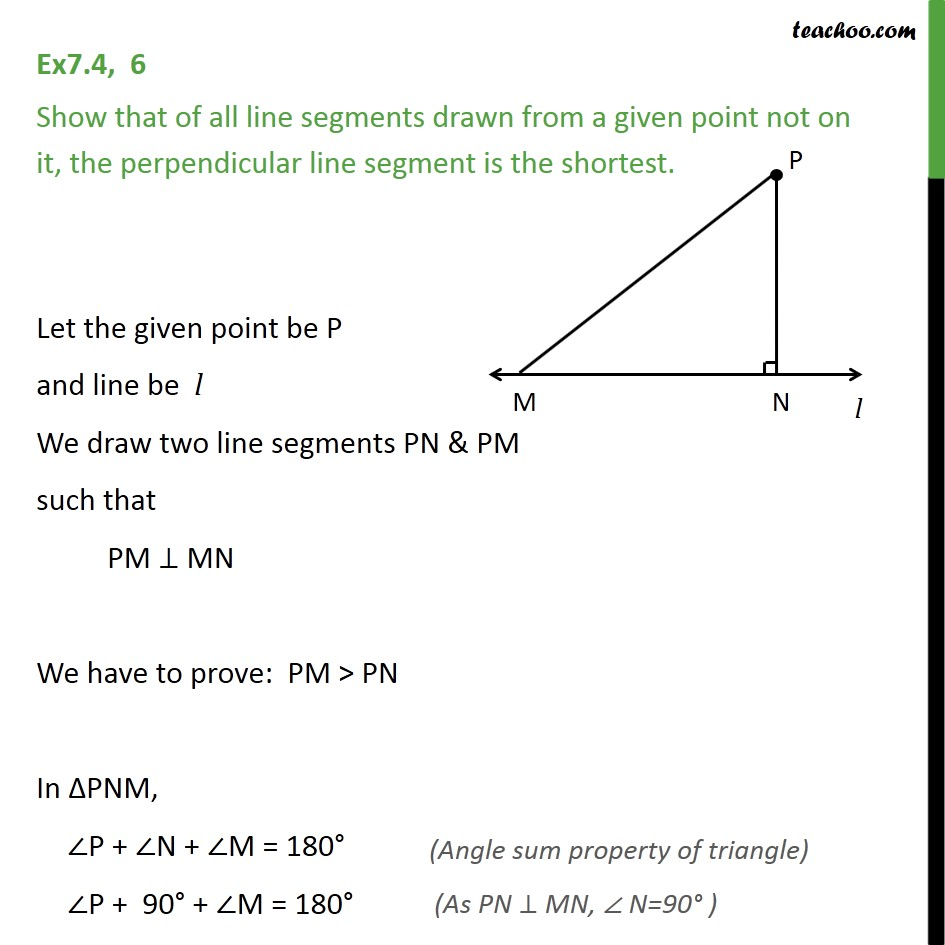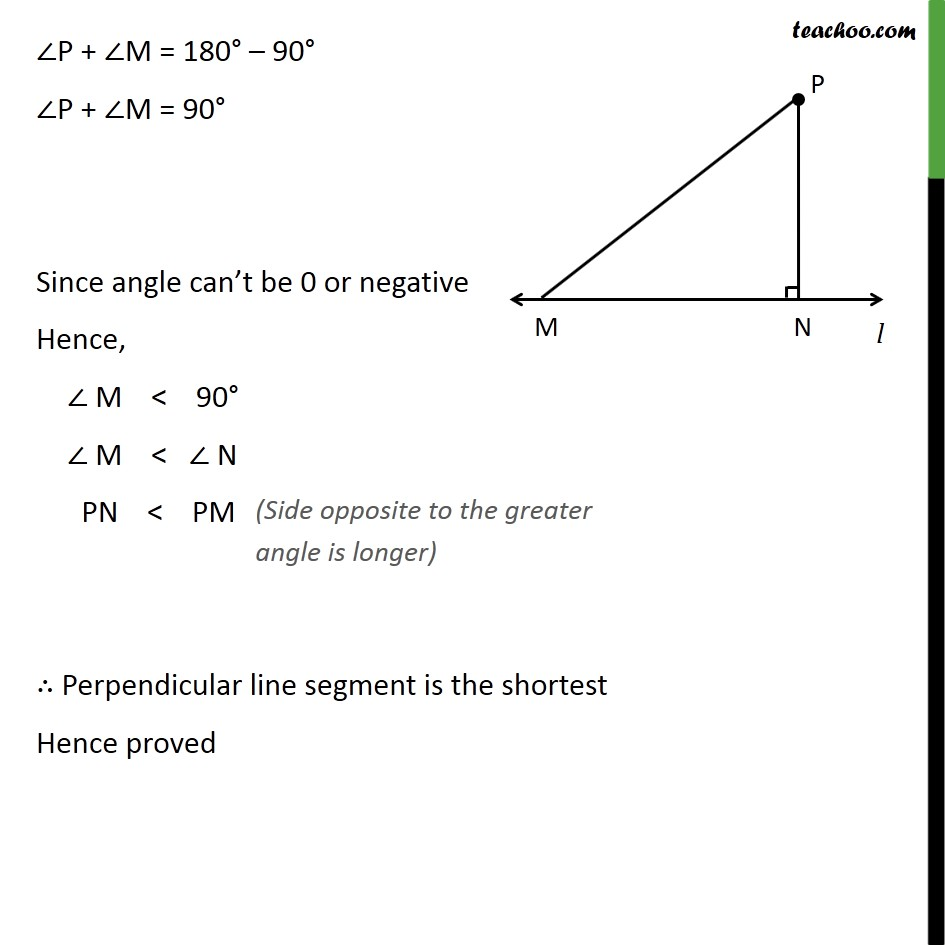Angle opposite to longer side

Chapter 7 Class 9 Triangles
Serial order wiseLearn in your speed, with individual attention - Teachoo Maths 1-on-1 Class

### Transcript

Ex7.4, 6 Show that of all line segments drawn from a given point not on it, the perpendicular line segment is the shortest. Let the given point be P and line be l We draw two line segments PN & PM such that PM ⊥ MN We have to prove: PM > PN In ΔPNM, ∠P + ∠N + ∠M = 180° ∠P + 90° + ∠M = 180° ∠P + ∠M = 180° – 90° ∠P + ∠M = 90° Since angle can’t be 0 or negative Hence, ∠ M < 90° ∠ M < ∠ N PN < PM ∴ Perpendicular line segment is the shortest Hence proved